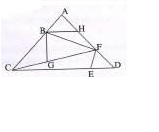## WBCS MAIN PAPER VI : MATHEMATICS - AVERAGE AND SUMMATION

1.The average of first ten prime numbers which are odd is (A) 12.9 (B) 13.8 (C) 15.8 (D) 17

2.The average of first 10 even numbers is (A) 18 (B) 22 (C) 9 (D) 11

3.The average age of three boys is 15 years and their ages are in proportion 3: 5:7. What is the age in years of the youngest boy?

(A) 15 (B) 9 (C) 18 (D) 21

4.The average of first 10 natural numbers is (A) 5 (B) 5.5 (C) 6.5 (0) 6

5.If the average marks of three batches of 55, 60 and 45 students respectively is 50, 55, 60, then the average marks of all the students is

(A) 53.33 (B) 54.68 (C) 55 (D) None of the above

1.Average age of 8 sons of a family is 12 years. Average age of the sons together with their parents is 21 years. If the father is older than the mother by 8 years, then the age of the mother is

(A) 48 years       (B) 53 years              (C) 60 years    (D) 62 years

2.The average weight of 8 persons increases by 2.5 kg when a new person comes in place of one of them weighing 65 kg. What might be the weight of the new person ?

(A) 76 kg          (B) 5 kg      (C) 85 kg      (D) None of the above3.What is the number of triangles in the following figure ?

(A) 10  (B) 9       (C) 11            (D) 12

4.If the average of a, b, c is 15, the average of b, c, d is 12 and the average of a, d is 13, the value of d is

(A) 34              (B) 30              (C) 24       (D) 10

1.There are five positive observations. Average of the first three observations is 8 and that of the last three observations is 6. If the average of all five observations is 6, what is the third observation ?

(A) 10              (B) 8       (C) 12            (D) 14

1.The mean of 19 observations is 4. If one more observation of 24 is added to the data, the new mean will be

(A) 5   (B) 6   (C) 4   (D) 7

2.The average of three numbers is 28. The first number is half of the second and the third number is twice the second. The third number is

(A) 48              (B) 18              (C) 24              (D) 36

3.The average weight of x, y, z is 45 kg. If the average weight of x and y be 40 kg and that of y and z be 43 kg, then the weight of y is

(A) 20 kg          (B) 26 kg        (C) 31 kg           (D) 17 kg

1.The average of first 100 natural numbers is-

(A) 50        (B) 50.5      (C) 51              (D) 51.5

2.A man bought 5 shirts at Rs. 450 each, 4 trousers at Rs.750 each and 12 pairs of shoes at Rs. 750 each. The average expenditure per article is :

(A) Rs. 678.50        (B) Rs. 800           (C) Rs. 900      (D) Rs. 1,000

3.The average score of 10 students in a test is 25. The lowest score is 20. Then the highest score is at most-

(A) 30        (B) 70         (C) 75       (D) 100

1.If the average of first 21 natural numbers is 11, average of first 20 natural numbers will be

(A) 9   (B) 9.5             (C) 10       (D) 10.5

2.Out of 11 numbers average of first 6 numbers is 8.5 and the average of last six numbers is 6.5. If the average of 11 numbers is 8, the sixth number is

(A) 1       (B) 2              (C) 3      (D) 4

3.Average of five consecutive Odd numbers is 15. The least number is

(A) 11              (B) 13              (C) 15              (D) 17

4.The average of two numbers is xy. If one number is X, the other number is

(A) y/2 (B) y        (C) (2xy - x)               (D) x(y - 1)

5.The mean of five numbers is 18. If one number is excluded, their mean is 16. The excluded number is

(A) 25        (B) 26         (C) 27              (D) 30

1.The average marks of boys in a class is 52 and that of girls is 42 . The average marks of boys and girls combined is 50.  What is the percentage of boys in the class ?

(A) 70%           (B) 75%        (C) 80%              (D) 82%

2.The average of first 51 natural numbers is 26. What is the average of first 50 natural numbers ?

(A) 24              (B) 24.5           (C) 25        (D) 25.5

3.The mean proportion between 9 and 16 is

(A) 12.5       (B) 12       (C) 5   (D) None of the above

4.Average weight of some students is 55 kg.; of them average weight of 20 students is 60 kg. and average weight of remaining students is 51 kg. What is the number of students ?

(A) 30       (B) 35      (C) 40         (D) 45

5.A student wrote 54 instead of 45 by mistake. As a result he got the average of 5 numbers as 52. What is the correct average of the numbers ?

(A) 48    (B) 48.8      (C) 50      (D) 50.2

Shopping Cart